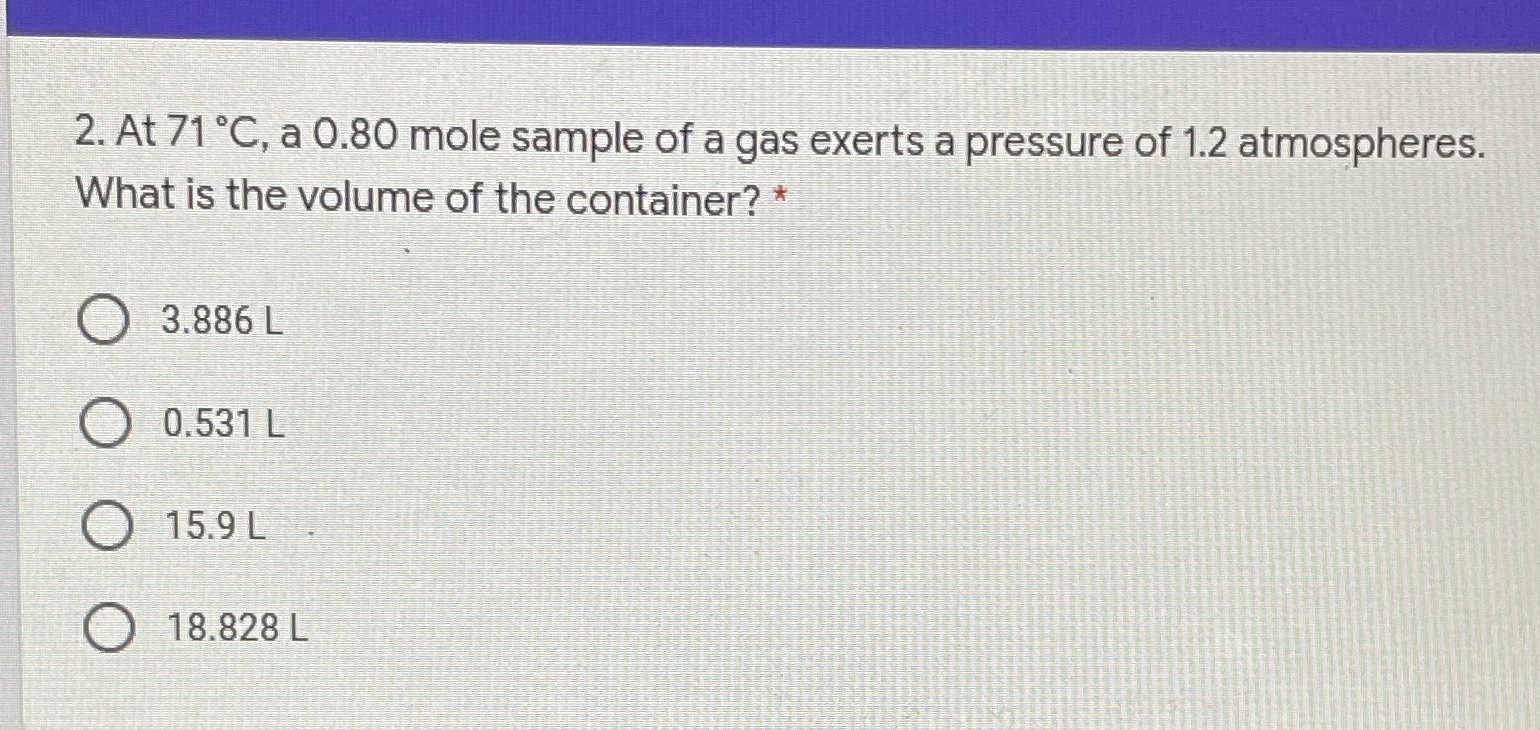### Still have math questions?

Arithmetic
Question2. At $$71 ^ { \circ } C ,$$ a $$0.80$$ mole sample of a gas exerts a pressure of $$1.2$$ atmospheres. What is the volume of the container? *

$$3.886 L$$

$$0.531 L$$

$$15.9 L$$

$$18.828 L$$

$$18.828 L$$